Go back to  'Time'

Tim: Mom, why is my favorite cartoon not coming on the TV?

Mom: Son, you have been late by 1 hour to watch your favorite cartoon.

Tim: No mom, I saw the cartoon yesterday at this time.

Mom: Son, your cartoon's time is 2:00 P.M. and right now the clock is showing 3:00 P.M.

Tim: Dad! Teach me how to read time from the clock. I don’t want to miss my cartoon next time.Do you also want to learn how to read the clock like Tim?

Come, let us learn in detail about Reading and Representation of Time.

## Lesson PLan

 1 Reading and Representation of Time 2 Tips and Tricks 3 Solved Examples on Reading and Representation of Time 4 Important Notes on Reading and Representation of Time 5 Interactive Questions on Reading and Representation of Time

## Reading and Representation of Time

### Definition of Time

Time is a concept that is at once measurable and also infinite. It all depends on where you set the starting point for your measurement.

The universe is billions of years old, yet we don’t measure time in terms of the age of the universe, rather we measure time in more meaningful units.

Before setting off into the world of reading a clock face, one must understand to differentiate certain activities based on the time of day. This helps them internalize the flow of time.Reading a clock is a skill that’s easy to master with a little time and effort. You will be reading clocks with ease.
First of all, every day has 24 hours.
The clock is divided into 12 sections with each section designated with a number. Every clock shows numbers and has two ‘hands’, which are called the hour hand and the minute hand.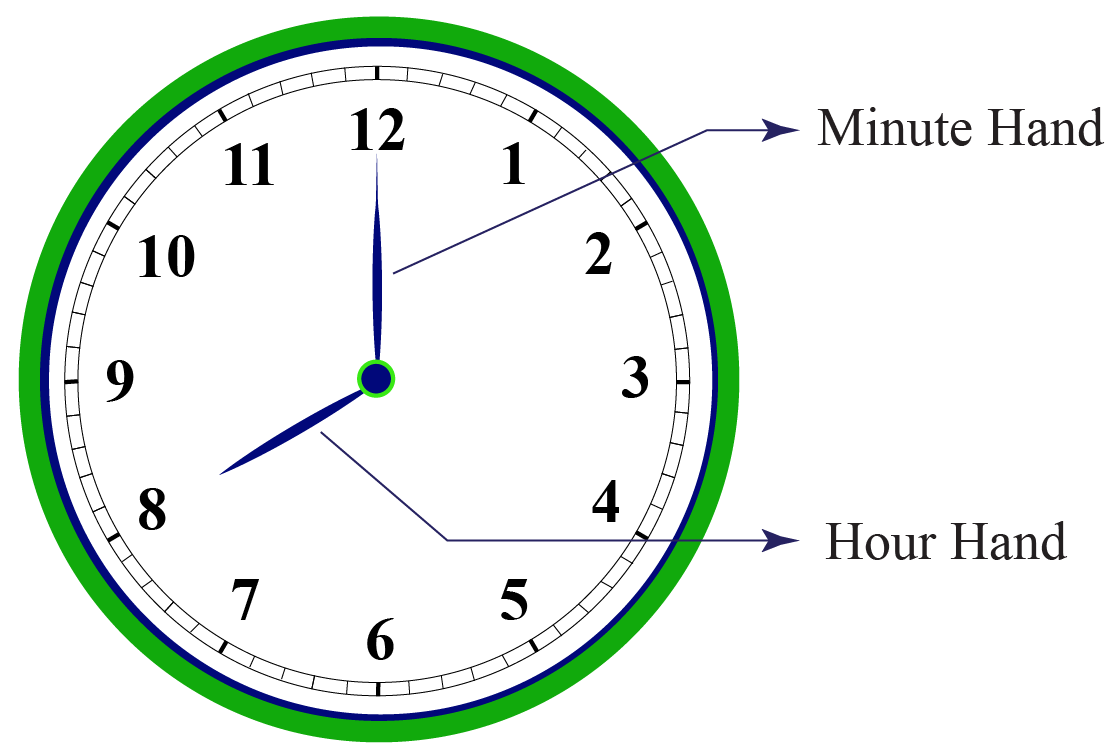The small hand marks hours and the long hand marks minutes. The hands point to a number and the combination of the hour and minute hand facing numbers give us the time.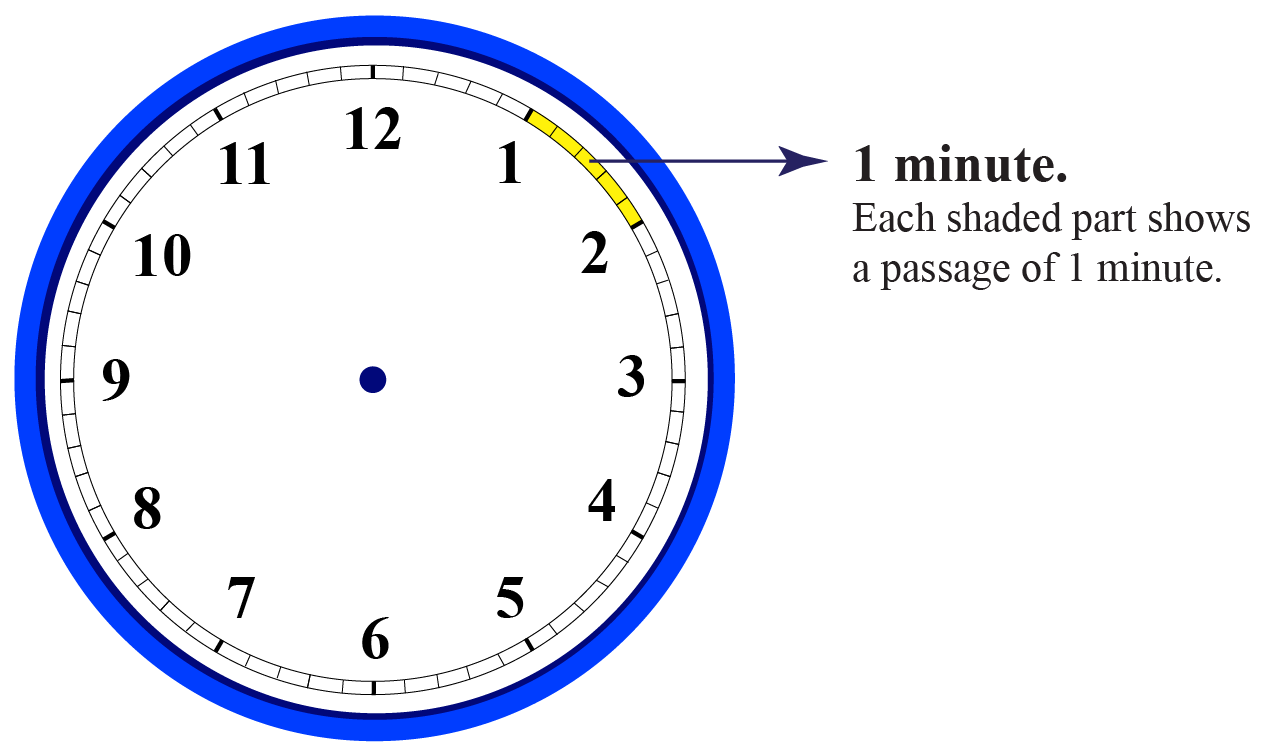The sections between numbers are divided into 5-minute segments. The minutes start with 0 at 12, and for every number thereafter, the minute hand represents 5 minutes.

Let’s start with a basic example. Look at the hour hand in the picture below. It points at 9, so the hour is 9 and looking at the minute hand which points at 12 gives us the time 09:00 or 9 o’clock.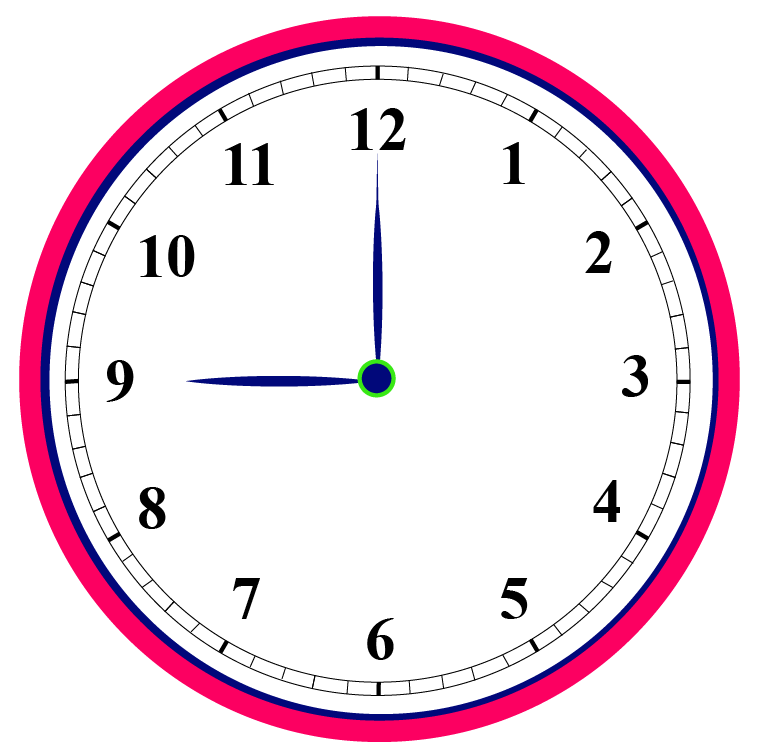### How Do You Calculate Time?

Let’s raise the level a little bit, observe the image below.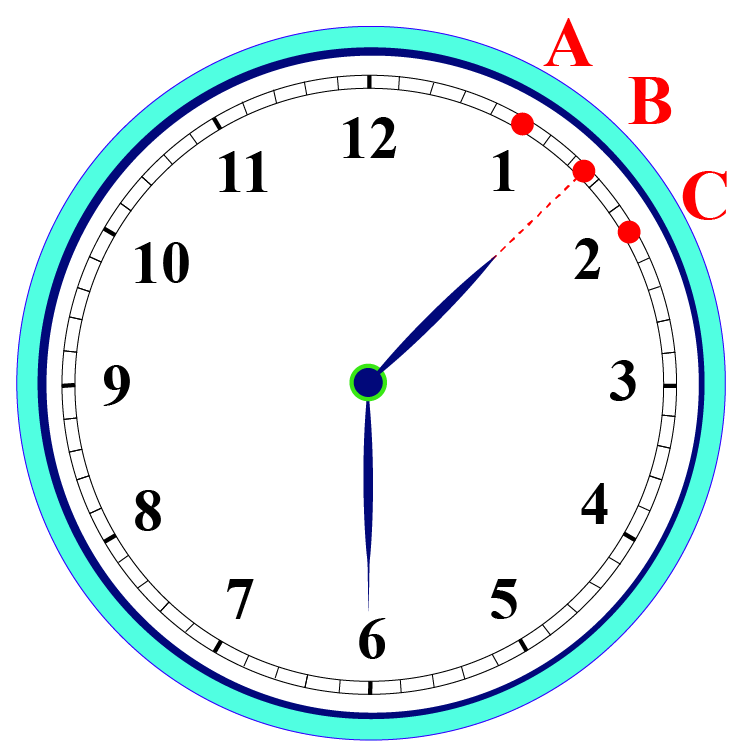With every minute that passes, the hour hand would have covered some of the distance between 1 and 2.

When the minute hand would have started at 12 and completed one circle back to 12, the hour hand would have gone from 1 to 2.

Since each small section comprises 5 minutes, the time that has passed is $$12\times 5 = 60$$ minutes.

So, if the minute hand were at four instead of six, the minutes passed would be $$4\times 5=20$$ minutes.

So, this makes it clear that 1 hour is equal to 60 minutes. From 12 to 1, 2, 3 and so on till 11 and then 12, the hour hand crossing 1 number represents an hour.

The movement of the minute hand from one number to the other on the clock shows a movement of 5 minutes.

An additional really thin hand on the clock that is rotating really fast? It represents seconds. Every minute consists of 60 seconds. Given below is a table on time conversion:

 1 Day 24 Hours 1 Hour 60 Minutes 1 Minute 60 Seconds

## Represent Time In 12 Hours Clock

The time of day written in the 12-hour clock runs from 1 am to 12 noon and then 1 pm to 12 midnight.

From 12 o’clock at midnight to 11:59 in the morning, the time is referred to as a.m.
From 12 noon to 11:59 in the night, the time is referred to as p.m., AM stands for Ante Meridiem (before noon), and PM stands for Post Meridiem (afternoon).

Ante-meridiem means before the Sun has crossed the meridian line and post-meridiem means after the Sun has crossed the meridian line.

### Example

In the 12-hour format, we need to take the help of AM and PM to describe the time properly. So, if it is 20 minutes past 9’oclock in the morning, it is 9:20 AM. But at the same time in the evening, it would be described as 9:20 PM.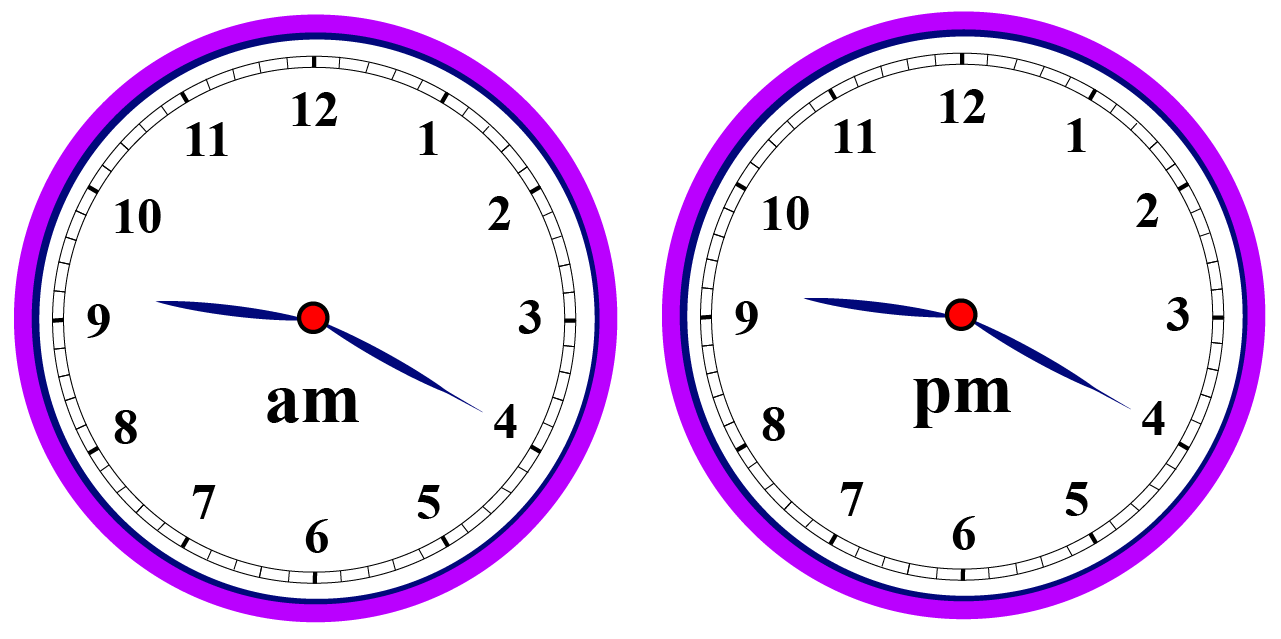The major disadvantage of the 12-hour format is the higher chances of miscommunication. It may so happen that one confuses AM with PM. That's why we have the 24-hour format. The 24-hour clock format is commonly used for time representation in the military, railway stations, airports, etc.

## Represent Time In 24 Hours Clock

The time of day written in the 24-hour clock uses the number 00:00 to 23:59 (midnight is 00:00)

In 24-hour format, time is described as the 24-hour period. So, 9.20 AM would be written as 0920 hours, whereas 9.20 PM would be described as 2120 hours.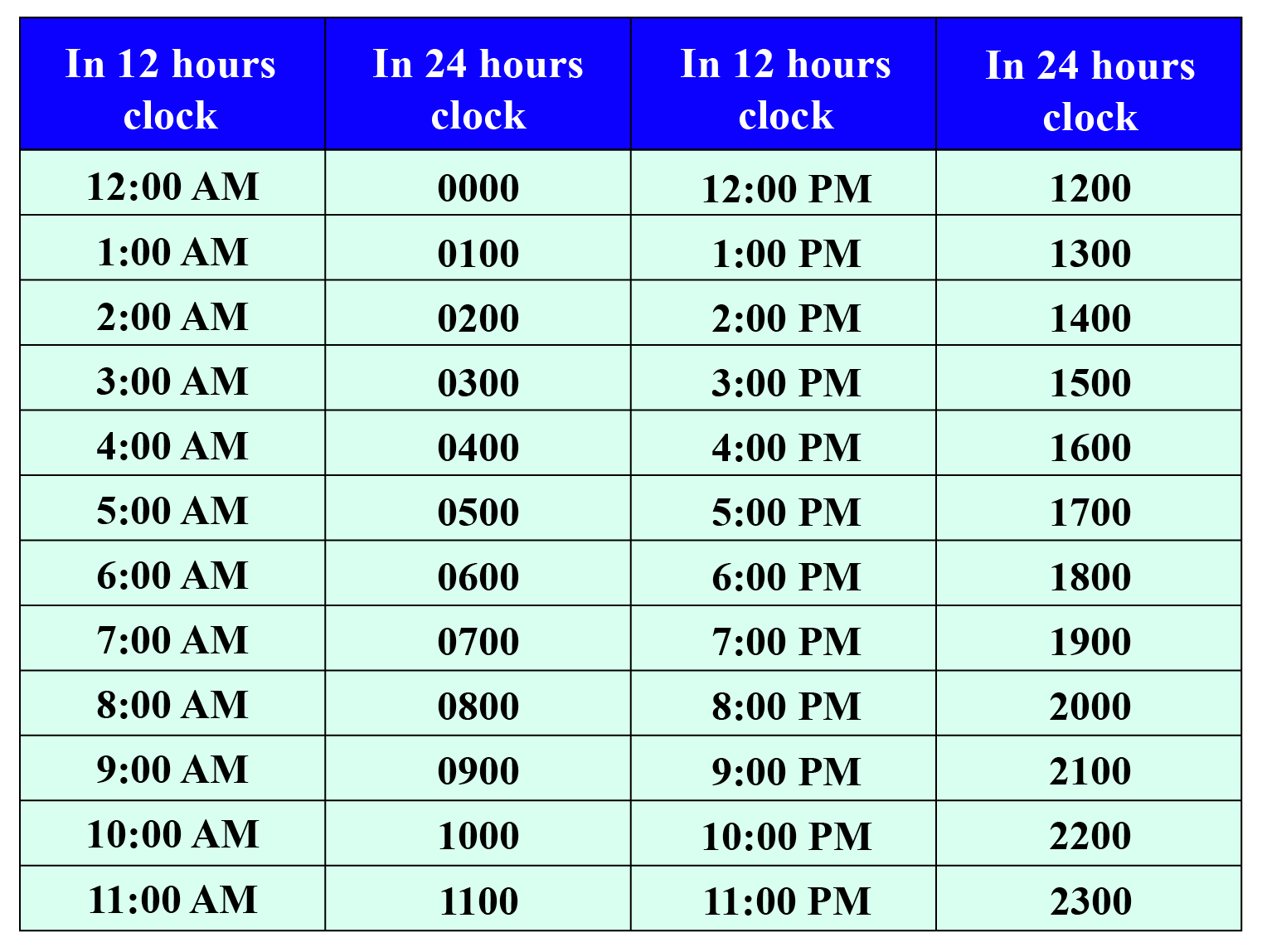Example

Consider 15:30

This is 15 hours from the beginning of the day, which means it is past the morning and is the afternoon. Taking off the 12 hours in the morning gives 3 hours, so the time is 3:30 P.M.Tips and Tricks

• For the 24-hour clock, we simply add 12 to the hours of the 12-hour clock and remove P.M. For example 10:00 P.M. in the 12-hour clock is $$(10+12=22)$$ 22:00 in the 24-hour clock.
• And we can simply subtract 12 from the hours of the 24-hour clock and add P.M. i.e., 22:00 in the 24-hour clock is $$(22-12=10)$$ 10:00 in the 12-hour clock.

## Calculating Time Elapse

Time elapsed is the amount of time that passes from the beginning of an event till the end of that event.

Time elapsed = Starting Time + Time Taken

The calculator below will calculate the time elapsed from the starting time to the end time. Enter the starting time and the end time and press the "Go button".

## Solved Examples

 Example 1

Max, Rosy, and Jose go to school every day at 7:00 A.M. Their school bus takes a half-hour to reach the school.At what time does the school bus reach school?

Solution

We know that half-hour = 30 Minutes

Time elapsed = Starting Time + Time Taken

= 7:00 + 0:30 A.M.

= 7:30 A.M.

 $$\therefore$$ School bus reaches at 7:30 A.M. every day.
 Example 2

Andrea needs help with the following conversions. Can you help her?

1. $$3$$ minutes $$15$$ seconds into seconds.

2. $$4$$ hours $$30$$ minutes into minutes.

3. $$2$$ days $$8$$ hours into hours.

Solution

1. We know that $$1$$ minute = $$60$$ seconds

therefore, $$3$$ minutes $$15$$ seconds

=$$(3\times 60)$$ seconds + $$15$$ seconds
=$$180$$ seconds + $$15$$ seconds
=$$195$$ seconds

2. We know that $$1$$ minute = $$60$$ seconds
therefore, $$4$$ hour $$30$$ minutes

=$$(4\times 60)$$ minutes + $$30$$ minutes
=$$240$$ minutes + $$30$$ minutes
=$$270$$ minutes

3. We know that $$1$$ day = $$24$$ hours
therefore, $$2$$ days $$8$$ hours

=$$(2\times 24)$$ hours + $$8$$ hours
=$$48$$ hours + $$8$$ hours
=$$56$$ hours

 $$\therefore$$  1. 195 seconds 2. 270 minutes 3. 56 hours
Time
Kg | Worksheet 1
Time
Kg | Worksheet 2

More Important Topics
Numbers
Algebra
Geometry
Measurement
Money
Data
Trigonometry
Calculus